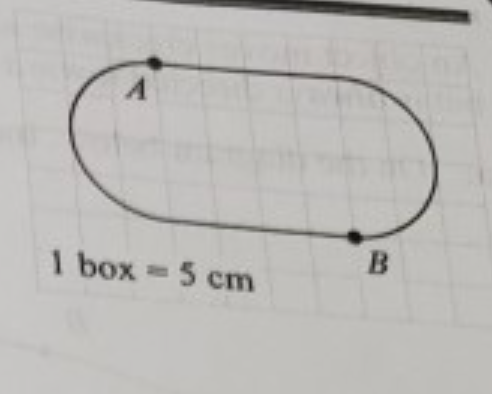# Problem: Some students used two straight segments and two half circles to make a track for a toy car, shown in the scale diagram at right. Each box on the diagram is 5 cm on a side.The car moves clockwise around the track at a constant speed. It takes the car 8 s to go around the track once.a. Determine the speed of the car. Show your work.b. Consider the interval that begins when the car passes point A and ends when the car passes point B (halfway around the track).i. On the diagram at right, draw an arrow to represent the direction of the average velocity over this interval. Explain your reasoning.ii. Determine the magnitude of the average velocity of the car over this interval. Show your work.iii. On the diagram at right, draw an arrow to represent the direction of the average acceleration over this interval. Explain your reasoning.iv. Determine the magnitude of the average acceleration of the car over this interval. Show your work.

###### FREE Expert Solution

Speed is calculated from:

$\overline{){\mathbf{S}}{\mathbf{p}}{\mathbf{e}}{\mathbf{e}}{\mathbf{d}}{\mathbf{=}}\frac{\mathbf{D}\mathbf{i}\mathbf{s}\mathbf{t}\mathbf{a}\mathbf{n}\mathbf{c}\mathbf{e}}{\mathbf{T}\mathbf{i}\mathbf{m}\mathbf{e}}}$

a.

We need to find the total distance of the track.

We've been told that 1 box = 5 cm

Therefore, the length of the two segments is given by:

L = (2)(5)(4) = 40 cm

The length of two half circles is found from the circumference of a circle.

Circumference,

$\overline{){\mathbf{C}}{\mathbf{=}}{\mathbf{\pi }}{\mathbf{D}}}$, where D is the diameter.

D = (5)(4) = 20 cm

98% (299 ratings)###### Problem Details

Some students used two straight segments and two half circles to make a track for a toy car, shown in the scale diagram at right. Each box on the diagram is 5 cm on a side.The car moves clockwise around the track at a constant speed. It takes the car 8 s to go around the track once.

a. Determine the speed of the car. Show your work.

b. Consider the interval that begins when the car passes point A and ends when the car passes point B (halfway around the track).

i. On the diagram at right, draw an arrow to represent the direction of the average velocity over this interval. Explain your reasoning.

ii. Determine the magnitude of the average velocity of the car over this interval. Show your work.

iii. On the diagram at right, draw an arrow to represent the direction of the average acceleration over this interval. Explain your reasoning.

iv. Determine the magnitude of the average acceleration of the car over this interval. Show your work.